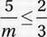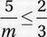# SAT Math Multiple Choice Question 624: Answer and Explanation

### Test Information

Question: 624

9. If, where m > 0, what is the least possible value of m?

• A. 6.5
• B. 7
• C. 7.5
• D. 8

Explanation:

C

Original inequality:Multiply by 3m (since m > 0, we don't "flip" the inequality):

15 ≤ 2m

Divide by 2:

7.5 ≤ m

Therefore, the least possible value of m is 7.5.